A sharp knife has smaller area at its edge than a blunt knife. When a force is applied on the knife, The pressure exerted by the edge of sharp knife is more than the pressure exerted by the blunt knife during cutting. Due to this reason, cutting with a sharp knife is easier than cutting with blunt knife.

When the area is increased by keeping the magnitude of force constant, the pressure exerted is decreased because pressure is inversely proportional to the area of surface that receives the force.

When a man stands with one foot the area of surface where the force is applied is decreased and as a result the pressure is increased because keeping the force constant, the pressure is inversely proportional to the area of surface that receives the force.

1. The pressure, the force applied is directly proportional to its magnitude, that receives the area.

P α F……….. (i)

2. The force that gives pressure is inversely proportional to the area that receives the force.
P α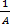………….. (ii)
From equation (i) and (ii)
P α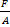P = K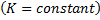If 1 N force is applied in 1 square meter area, then the pressure will be 1N/m2 and thus, k=1.

Mass of a man (m) = 60 kg

Acceleration due to gravity (g) = 10 m/s2

Weight of the man (F) = 600 N

Area occupied by one foot (A) = 150 cm2 =0.015 m2

Pressure exerted (P) =?

P ==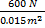=40,000 N/m2

This pressure exerted by the man on the ground when he stepped with one foot is 40,000 Pa.

Here,

Mass of Anupama (m) = 30 kg

Weight of Anupama (f) = mg = 30 kg × 10 m/s2= 300N

Surface area of a sandal (A) = 150 cm2 =0.015 m2

Pressure exerted (P) =?

P ==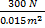=20,000 N/m2

This pressure exerted by the sandal on ground when Anupama stands on one foot is 20,000 Pa.

The pressure is defined as “the force acting perpendicularly per unit area”. The pressure is defined as the perpendicularly applied force on a unit area. The SI unit of pressure is N/m2. It is also called Pascal (pa).

Following are the examples show us the extensive use of pressure in our daily life:

1. Food is cooked faster in a pressure cooker than an open pot due to high air pressure.
2. Among the taps of same size and connected to a common pipeline in a house, the flow of water from the tap on the lower floor is more than on the higher floor.

The two factors that affect the pressure are as follows:

1. The magnitude of force(f)
2. The surface area on which force is extended.

The pressure developed in 1 meter area when 1N of force is applied is called 1 Pascal of pressure.

1 pa =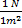The two aspect of our daily life where pressure theory is used are: 1. Application of pressure cooker to cook our food. 2. The flow of water is highest at the tap connected at the lowest level from the tank than the subsequent taps above it.
As we know pressure is inversely proportional to the area on which the force is applied. When area is increased (keeping the force constant) the pressure is decreased as the same foce now has to act on large area so force gets dispersed.
When a man stands on one foot the surface area on which is force is acting is smaller compared to when he is standing on two foot. So, as the area is decreased the pressure applied is increased (because force is inversly proportional to the area). So, A man exerts more pressure under his foot when he stands with one foot than when he stands on two foot.
As the knife gets sharper the surface area of the knife's cutting edge have very less surface area. When the surface area is reduced the pressure applied with the same amount of force is increased as pressure is inversely proportional to the surface area. So the knife with sharp edge (having less surface area) cuts easily tahn the blunt ones (having more surface area).
The lady wearing pointed heel will exert more pressure because we know pressure is inversly proportional to the surface area and the area of the pointed is less than the flat shoe.

Here, Area of the base (A) = 10 cm x 18 cm

= 0.1 m x 0.18 m

= 0.018$$m^{2}$$

Depth of water in the vessel (h) = 4 cm = 0.04 m

Density of water (d) =1000 kg/$$m^{3}$$

∴Force on the base (F) =?

We know that

P = dgh

= 1000 $$\times$$ 10$$\times 0.04 = 400Nm^{-2}$$

Again we that

$$P =\frac {F}{A}$$

∴ F =P ×A

= 400 × 0.018

=7.2N

Here, Mass of the girl (m) = 30 kg

Surface area of single shoe (A) = 100 cm2

= 1 ×10-4m2

∴ Weight(F) = mg

= 30 ×10 = 300N

We know that,

p=$$\frac{F}{A}$$

=$$\frac{300}{1 ×10^{-4}}$$

= 3 ×106pa

Hence, pressure exerted on the ground is 3 × 106pa.